# Why FP Matters - Part 2½ 🍰Picture by Annie Spratt

Slicing complexity with Higher-order Functions

# A Quick Recap

In the last article Why FP Matters - Part 2 🎈: Higher-order functions, we focussed on the basics of Higher-order functions.

1. We learned about what it means for functions to be first-class.
2. We were introduced to Partial application.
3. Lastly, we tasted some curry.

Well, now it is time for something sweet! 😋

If you don't know what I am talking about, this is a series of articles. To have better context, you might want start here - Why FP Matters - Part 1 🐘 : A brief introduction

# What we will cover in this post?

As I promised, there will be more code. There will be around 48 lines of it. Yes, 48 lines with white space and execution samples included 🙂. We will write code to perform the below

1. Add all numbers in a list
2. Multiply a list of numbers
3. Check if all values in a list are `True`
4. Check if any value in a list is `True`
5. Reconstruct a list and return as is
6. Transform all the values in a list
7. Add all the numbers in a matrix
8. Add all the numbers in tree
9. Collect values from the tree in a list
10. Transforming all the values in a tree

We will achieve all of this with the help of small yet powerful reusable functions. This should help us realize the power of higher-order functions. I also promise you a BONUS 🎁 at the end of the blog, for all you folks who are bored of looking at all the Haskell code.

If you are curious, below is a teaser of what the code will look like in the end. Let's get started!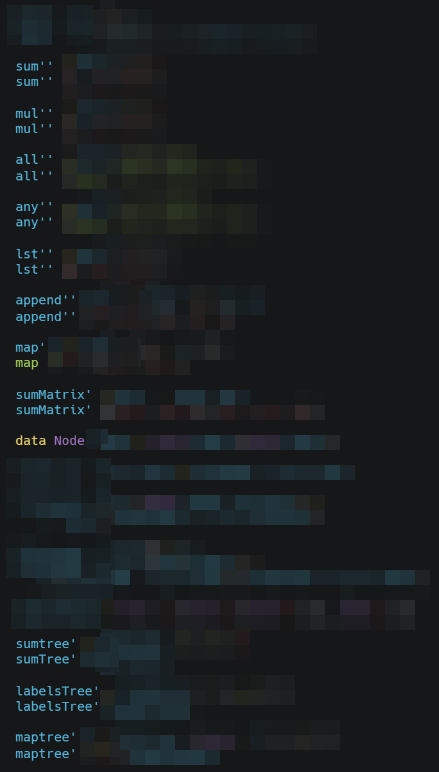# Whole Sum RecursionPicture by Natallia Nagorniak

Let's say that you serve someone some morsels of food on a plate. Do you instruct them about how to pick each morsel and eat it? No, you just tell them to enjoy their food. This works for most adults. Wouldn't it be nice if we could do the same with our programs? We could partly, but computers take things too literally. We need to tell them when to stop.

For example, below is a function that receives a list of numbers as input, and we want to return the sum of all the numbers in the list. One version of the function would look like the below.

Note: `head` is a function available in Haskell that returns the first element in the list, while `tail` is a function that returns everything except the first element in the list.

`sum' xs = if xs == []  then    0  else    (head xs) + (sum' (tail xs))`

This works.

• Every time sum is called with an input, we check if the list is empty. This is the terminating condition.
• If the input is an empty list, we simply return `0` (zero).
• Else, we take the first element, and add it to the sum of the rest of the list.
`-- below evaluates to 6sum' [1, 2, 3]`

Since we are not relying on global state or modifying it, and we are dependent only on the input to the function, our programs have referential transparency. We can use the substitution method to prove that our program works. We simply replace the definition of the function as applicable at each step.

• `sum' [1, 2, 3]`
• `(head [1, 2, 3]) + sum' (tail [1, 2, 3])`
• `1 + sum' [2, 3]`
• `1 + (head [2, 3]) sum' (tail [2, 3])`
• `1 + 2 + sum' `
• `1 + 2 + (head ) sum' (tail )`
• `1 + 2 + 3 + sum' []`
• `1 + 2 + 3 + 0`
• `1 + 2 + 3`
• `1 + 5`
• `6`, which is returned as our final result.

Note: `sum'` returns a `0` (zero) for an empty list

All this would have been good for us a few weeks back. But we've learned some Haskell along the way. We know that it has some sweet sugary magic.

So, by applying pattern matching we can get rid of the `if-then-else` and the below

`sum' xs = if xs == []  then    0  else    (head xs) + (sum' (tail xs))`

can be re-written as

`sum' [] = 0sum' xs = (head xs) + (sum' (tail xs))`

Clean! Oh, you don't agree. Let's put a potion of the destructuring in there too. This will get rid of the need to use `head` and `tail`. We will be self reliant.

`sum' [] = 0sum' (x:xs) = x + sum' xs`

This version works exactly the same way as we worked out above. How do we read this? Simple

• If you get an empty list return a `0` (zero)
• Otherwise, detach the first element into the variable `x`, leaving the other elements in the list assigned to `xs`
• Add `x` to the `sum'` of the rest of the elements `xs`

Well, I hope you are happier now. We dropped a lot of characters with that one. We could follow this pattern and write more useful functions.

`-- multiply all numbers in the listmul' [] = 1mul' (x:xs) = x * mul' xs--  return reconstructed listlst' [] = []lst' (x:xs) = x : lst' xs`

No? You are not happy? Well alright then. I think there is no pleasing you today. Let's try refactor. Let's try to fold ...

# 🧬 FoldPicture by Monika Grabkowska

We will attempt to refactor the functions and see if there are any reusable parts. But first, let's make use of the charming operators as function trick. So, the below

`sum' [] = 0sum' (x:xs) = x + sum' xsmul' [] = 1mul' (x:xs) = x * sum' xslst' [] = []lst' (x:xs) = x : sum' xs`

can be written as ...

`sum' [] = 0sum' (x:xs) = (+) x (sum' xs)mul' [] = 1mul' (x:xs) = (*) x (mul' xs)lst' [] = []lst' (x:xs) = (:) x (lst' xs)`

How did that help? If you look closely, the shape of the functions is similar.

• They all take in a list
• They return a default value for an empty list which varies based on the function
• They apply an aggregating function that takes in an element from the list and the output from the recursively called themselves on the rest of the list.
• This aggregating function is also different for each of the above functions.

Wouldn't it be nice, if we could pass in just the differences to special function and get the required behavior for each of our above functions? Let's define that as a higher-order function and call it `fold'`.

`fold' f a [] = afold' f a (x:xs) = f x (fold' f a xs)`

Now let's call `fold'` with `f` as `(+)`, `a` as `0` (zero) and a list of numbers. Now applying the substitution method.

• `fold' (+) 0 [1, 2, 3]`
• `(+) 1 (fold' (+) 0 [2, 3])`
• `(+) 1 ((+) 2 (fold' (+) 0 ))`
• `(+) 1 ((+) 2 ((+) 3 (fold' (+) 0 [])))`
• `(+) 1 ((+) 2 ((+) 3 0))`
• `(+) 1 ((+) 2 3)`
• `(+) 1 5`
• `6`, which is returned as our final result.

Note: `fold'` returns `a` for an empty list. Here `a` was `0` (zero).

We can define `sum'` in terms of `fold'` as below

`sum' xs = fold' (+) 0 xs`

I know, I know. You remember that we can do partial application and make this shorter. There you go.

`sum' = fold' (+) 0`

We can define our other methods using `fold'` too.

`mul' = fold' (*) 1lst' = fold' (:) []`

We can go on ...

`and' = fold' (&&) Trueany' = fold' (||) Falsecount' a n = a + 1len' = fold' count' 0`

Phew, that was a lot of code. I'm kidding of course. By the way, we are done with 14 lines of code and we have covered 4 out of 10 items that we listed as our targets above.

But is that the point of this all? To write less code? Well yes, and other things. What we have done here is

• Created a small but powerful higher-order function to increase re-usability of our
• The resulting code is smaller and less complex to read. It is a language of it's own.
• We can say, fold all numbers by adding them and start with the last number and a seed value `a`. That's it. The rest of the re-usable portion is abstracted within the `fold'` function.

Below is a simple visualization, to help you see how we extracted the differences.

• Remember that any a list of elements can be replaced with the sequence of elements interleaved with the `:` (cons) operator.
• We simply replace the `:` (cons) with the function `f` passed in as input to `fold`
• The empty list in the end gets replaced by the value of the input parameter `a`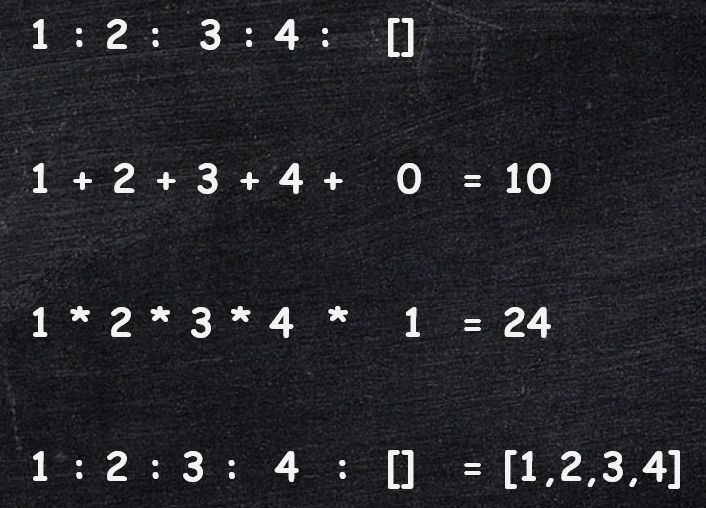Let's define one more function using `fold'` before we move on.

`append' xs ys = fold'  (:) ys xs`

Note: Here `f` is `(:)`, and `a` is `ys`.

Let's expand the below

• `append' [1, 2] [3, 4]`
• `fold' (:) [3, 4] [1, 2]`
• `fold' (:) [3, 4] (1 : 2 : [])`

Now replace `:` with `:` and `[]` with `[3, 4]`

• `(1 : 2 : [3, 4])`
• `(1 : [2, 3, 4])`
• `[1, 2, 3, 4]`

Voilà!

# 🎐 MapPicture by Wes Hicks

Wouldn't it be nice to have a function that transforms all the elements of a list. Perhaps we could use it to increment all the numbers in the list by `1`.

If we would have a function called `inc`

`inc' x = x + 1`

Then perhaps, we could use it as below and transform `[1, 2, 3]` to `[2, 3, 4]`.

`map' inc' [1, 2, 3]`

If we were to implement this method `map'` from scratch, it would look like the below.

`map' f [] = []map' f (x:xs) = (:) (f x) (map' f xs)`

On comparison with the earlier definitions of `sum'`, `mul'` and `lst'` above. It has a similar shape with just a couple of differences. In fact, if you compare it with `lst`, it is very similar in nature except for the below

1. it takes in a function `f`
2. it applies that method `f` before using `:` (cons) to combine the transformed element with the list. Hence, forming the transformed list.

There seems to be a possibility for using `fold'` here, but first we must refactor it a little.

First, let's look at the below function that

• takes in
• `f` the function to transform an element
• `x` an element
• `xs` a list
• and returns a new list
`fAndCons1 f x xs = (:) (f x) xsfAndCons2 f x    = (:) (f x)  -- partialfAndCons3 f x    = ((:) .f) x -- composefAndCons4 f      = ((:) .f)   -- partial`

using the above, we can change

`map' f [] = []map' f (x:xs) = (:) (f x) (map' f xs)`

to be

`map' f [] = []map' f (x:xs) = ((:) . f) (map' f xs)`

If you look closely, it is a pattern that can be replaced by `fold'`. Comparing it with `lst'`, it is clear here that every element `x` will first be transformed by `f` then prepended to the list.

• `((:) . f) x xs`
• `((:) (f x)) xs` , since `(f. g) x == f (g x)`
• `(:) (f x) xs`, `(:)` (cons) expects two inputs

using this, we can write `map'` in terms of `fold'`

`map' f = fold' ((:) . f ) []`

So, we have developed map, which is another generally useful function — applies any function `f` to all the elements of a list. And we did this using `fold'`. If you try to describe it in english, it says the we need to `fold'` or combine the elements in the list using `((:) . f )` i.e. transform then prepend to a list, and we start with an empty list `[].

Below is how we can use `map'` and use modularized transformations that live within separate functions.

`inc x = x + 1dbl x = x + xsqr x = x * xmap' inc [1, 2, 3] -- [2, 3, 4]map' dbl [1, 2, 3] -- [2, 4, 6]map' sqr [1, 2, 3] -- [1, 4, 16]`

Using what we have learned so far, the code to sum all the numbers in a matrix can be written as below. Note that we have chosen to represent the matrix as a list of lists.

`sumMatrix' = sum'' . map' sum''sumMatrix' [[1, 2, 3, 4], [1, 2, 3, 4]]`

Alright, we've come a long way and we have reaped the benefits of using higher-order functions to write short succint code to process lists. And we did all this from scratch using just functions and a little bit of syntactic sugar.

This all works well for lists, but what about other data structures? Can we write re-usable modules for them as well?

# 🎄 TreesPicture by Annie Spratt

Below is how you can define a data type for a tree in Haskell - `Node`. It takes in a value as the node `label` and it takes in a list of children. Each of the children are of type `Node`. It is a recursive definition.

`data Node label = Node label [Node label]`

Using the above, we can instantiate a tree as below.

• The root node has a label value `1` and it has two children
• The first child of the root node has a label value `2` and it has one child.
• The only child of the node with the label value `2` has a label value `4`, and it has no children.
• The seconds child of the root node has a label value `5` and it has no children.
`numberNodes =  Node 1 [      Node 2 [        Node 4 []],      Node 5 []]--       1--       +--       |--   2<----->5--   +--   |--   v--   4`

We can now define a function `foldtree'` function for our tree. This will be analogous to the `fold'` function that we defined for lists.

`foldtree' f g a node =  foldnode' f g a nodefoldnode' f g a (Node label children) =  f label (foldnodes' f g a children)foldnodes' f g a [] = afoldnodes' f g a (node:nodes) =  g (foldnode' f g a node) (foldnodes' f g a nodes)`

Tip: If you are trying to visualize this or use intuition, `f` is for combining the label of a node with a list of children `g` is for combining a child with it's siblings

Let's use `foldtree'` to define `sumtree'` a function that takes in a tree and returns a sum of all the label values.

`sumtree' = foldtree' (+) (+) 0`

We can use it to sum all the nodes in `numberNodes` as below

`sumTree' numberNodes -- returns 12`

If we use substitution to evaluate this we will end up with the below, then evaluate to `12`. If you are interested, the complete steps can be found here.

`(+) 1 (    (+) ((+) 2              ((+) ((+) 4 0)                  0))        ((+) ((+) 5 0)             0))`

Similarly, we can define `labelsTree'` to collect all the labels as below.

`labelsTree' = foldtree' (:) (++) []labelsTree' numberNodes`

`labelsTree numberNodes` will reduce to the below, and then evaluate to `[1, 2, 4, 5]`. Complete steps here.

`(:) 1 (    (++) ((:) 2              ((++) ((:) 4 [])                  []))        ((++) ((:) 5 [])             []]))`

For `labelsTree'`,

• `f` was the `:` (cons) operator
• `g` was the `++` (concat) operator, and
• `a` was `[]` (an empty list)

We could replace them in the above expression and get

`f 1 (    g (f 2              (g (f 4 a)                  a))        (g (f 5 a)             a))`

If you remember my tip earlier,

• `f` is applied on the label value, in this case `1`, `2`, `4`, and `5` to combine it with the reduction of the children.
• `g` is use to combine and reduce or fold the children.

foldtree'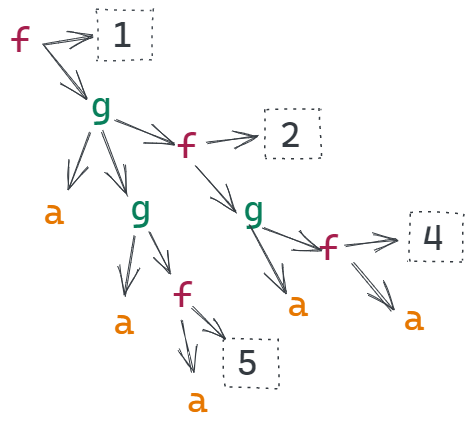sumtree'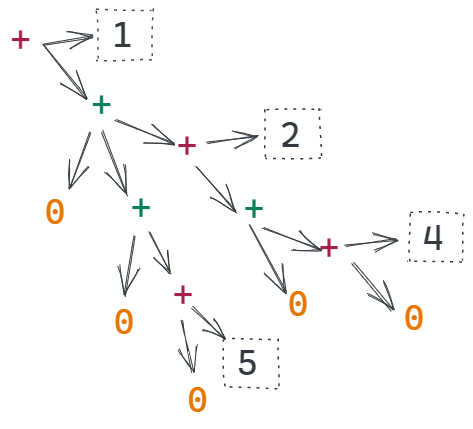labelstree'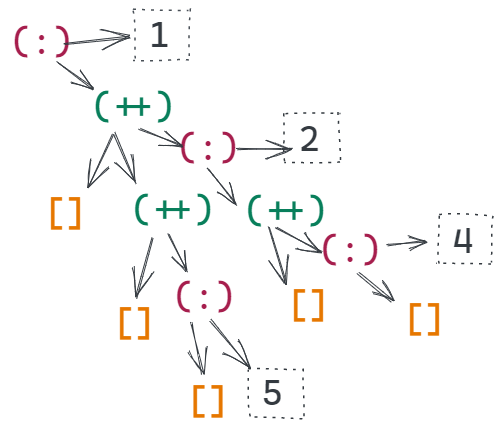And, finally we can use `foldtree'` to define `maptree'`, a function analogous to `map'`. Only, this one works on trees.

`maptree' f = foldtree' (Node . f ) (:) []maptree' (* 2) numberNodes`

# 📝 Conclusion

Final version of all the code that we saw in this post is as below. *raises curtain*

`fold'  f a [] = afold'  f a (x:xs) = f x (fold' f a xs)sum'' = fold' (+) 0sum'' [1, 2, 3, 4]mul'' = fold' (*) 1mul'' [1, 2, 3, 4]all'' = fold' (&&) Trueall'' [True, True, False, True]any'' = fold' (||) Falseany'' [True, True, False, True]lst'' = fold' (:) []lst'' [1, 2, 3, 4]append'' xs ys = fold' (:) ys xsappend'' [4, 5, 6] [1, 2, 3]map' f = fold' ((:) . f) []map' (* 2) [1, 2, 3, 4]sumMatrix' = sum'' . map' sum''sumMatrix' [[1, 2, 3, 4], [1, 2, 3, 4]]data Node label = Node label [Node label]foldtree' f g a node = foldnode' f g a nodefoldnode' f g a (Node label children) =  f label (foldnodes' f g a children)foldnodes' f g a [] = afoldnodes' f g a (node:nodes) =  g (foldnode' f g a node) (foldnodes' f g a nodes)numberNodes = Node 1 [Node 2 [Node 4 []], Node 5 []]sumtree' = foldtree' (+) (+) 0sumTree' numberNodeslabelsTree' = foldtree' (:) (++) []labelsTree' numberNodesmaptree' f = foldtree' (Node . f ) (:) []maptree' (* 2) numberNodes`

All this can be achieved because functional languages allow functions that are indivisible in conventional programming languages to be expressed as a combinations of parts — a general higher-order function and some particular specializing functions.

-- Why Functional Programming Matters by John Hughes

Which this is possible to an extent in some popular languages without higher-order functions by things like the strategy pattern, higher-order functions cut deeper and make the implementation much less noisy and easy to implement.

Once defined, such higher-order functions allow many operations to be programmed very easily.

-- Why Functional Programming Matters by John Hughes

We saw how defining, `fold'` and `foldtree'` could help us implement several aggregating (`sum'`, `sumtree'`, `sumMatrix'`, etc. ) and transforming function (`map'`, `maptree'`, etc) with ease and just a few characters.

Whenever a new datatype is defined, higher-order functions should be written for processing it. This makes manipulating the datatype easy, and it also localizes knowledge about the details of its representation.

-- Why Functional Programming Matters by John Hughes

The best analogy with conventional programming is with extensible languages — in effect, the programming language can be extended with new control structures whenever desired.

-- Why Functional Programming Matters by John HughesPicture by music4life

And now for the BONUS! You have been patient, I think you deserve more code, in the language that you understand. I have put together full working samples in 8 popular programming languages below for you to enjoy. Feel free to review!

Note:

• This is possible in other languages too, I just didn't get to them in time. And some, languages like Go, I avoided since they don't have generics so we won't get the kind of code reusability like other languages mentioned here. If you think you want to to try it in any other language that has higher-order function, please go ahead.
• As I mentioned in my previous posts, most popular languages have adapted functional aspects, so they will have methods like `fold'` and `map'` in-built or attached to list data structures, these examples just show that if required, we can take matter in our own hands. This is great, especially if we are going to define our own data structures.
• `fold'` is generally called `reduce`, `aggregate` or some such similar name.
• `map'` is alternatively as `select`.

If you think I can help you, of you think something is off, I am open to take requests and reviews 🙂. You can hit me up @ryanivandsouza or just comment on the GitHub gist page with the code samples.

1.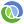Clojure
2.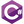C#
3.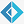F#
4.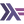Haskell
5.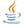Java
6.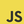JavaScript
7.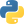Python
8.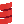Scala

# Summary

1. We defined a re-usable higher method `fold'` for processing lists.
2. We used `fold'` to define some aggregating functions for lists.
3. We went further and implemented a method `map'` using the `fold'` function.
4. Finally we defined a functions `foldtree` and `maptree'` for processing trees.

We saw how using functional programming helps is write re-usable components and how we can do a lot more with a lot less code.

If you are interested in dabbling around with Haskell, you have the below options.

If you don't want to get your hands dirty, that's fine too. We'll continue here with the next one in this series - Why FP Matters.

# Up Next

Why FP Matters - Part 3 🎩 - Lazy evaluation - Conjuring infinities: Things will get really lazy. There will be math, infinite lists, and a whole another level fun! We've just completed 8 out of 23 pages of the original content from the white paper by John Hughes1. We still have a lot to cover. See you next week.

Until then, have a happy holiday season! 🎅🏽🎄🤶🏽⛄🌟

For more fun stuff like this, click here to see a list of all my postsPicture by LoboStudioHamburg

1. Why Functional Programming Matters - http://www.cse.chalmers.se/~rjmh/Papers/whyfp.html